All You Need to Know About

# Index Formulas in Excel

Index Formulas in Excel are a very core part of your Excel toolkit. Learn about how to utilize the index function in Excel here as there are many creative ways in using this!

Here are the top things on what you can do with the Excel Index Formula:

## Sum a Range Using the INDEX Function

You can sum a range of values within a table using the INDEX function in Excel.  This is valuable when you want to extract key metrics from a table and put them in an Excel Dashboard.

To make this work you firstly need to start your Excel formula with the SUM function followed by the INDEX function.

So it will look something like this:  =SUM(INDEX(Array, Row_Num, Column_Num))

The Array will be your table of data, the Row_Num will be blank and the Column_Num will be the column number where you want to SUM the values.

When we dissect the formula (by highlighting the INDEX function and pressing F9) we can see that the following is happening: =SUM({8959;7840;7507;6690;5802;5487;3949;3836;3587;3210})

So in effect we are summing the array of values within the table.  See the example below that shows you how this is done.

STEP 1: We need to place first the INDEX function inside the SUM function.

## =SUM(INDEX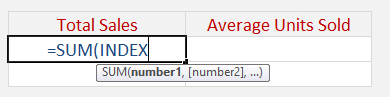The Index arguments:

## array

What is the range / table?

Reference the range of cells here that we want to get the values from:

## =SUM(INDEX(\$C\$12:\$E\$21,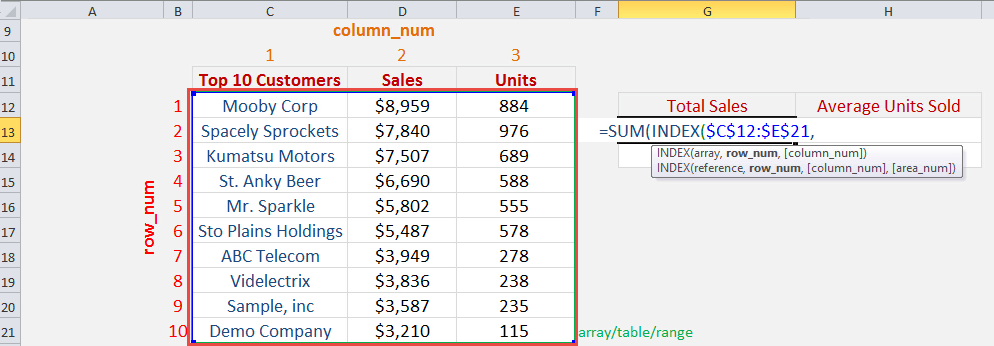## row_num

What is the row number we want to return?

We do not need to return the row, as we want to just sum all of the sales. Leave the row number blank:

## =SUM(INDEX(\$C\$12:\$E\$21,,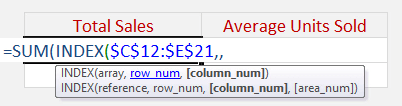## column_num

What is the column number we want to return?

Since we want the sales column, this is column number 2. So place in 2:

## =SUM(INDEX(\$C\$12:\$E\$21,,2))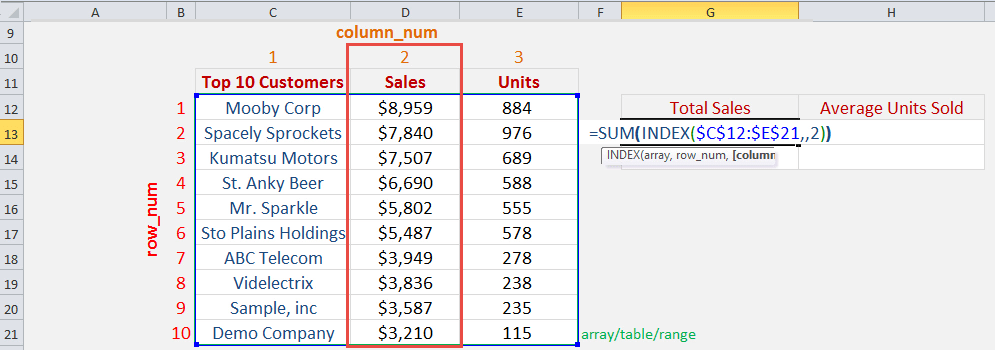Now you are able to get the Total Sales: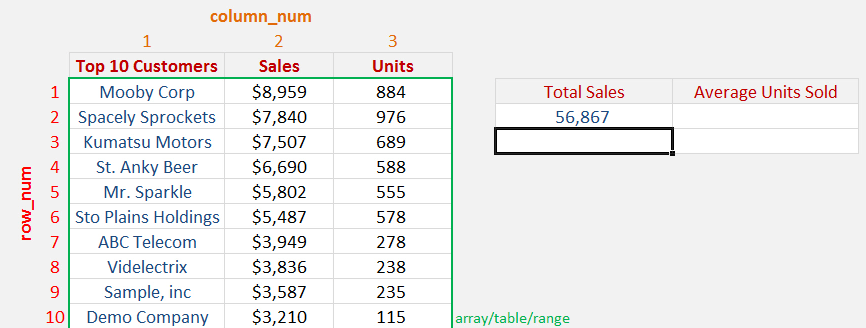STEP 2: Now let us try how we can use this with the AVERAGE function. We need to place first the INDEX function inside the AVERAGE function.

## =AVERAGE(INDEX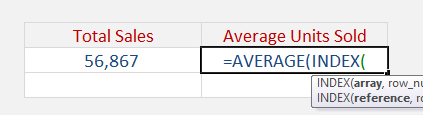The Index arguments:

## array

What is the range / table?

Reference the range of cells here that we want to get the values from:

## =AVERAGE(INDEX(\$C\$12:\$E\$21,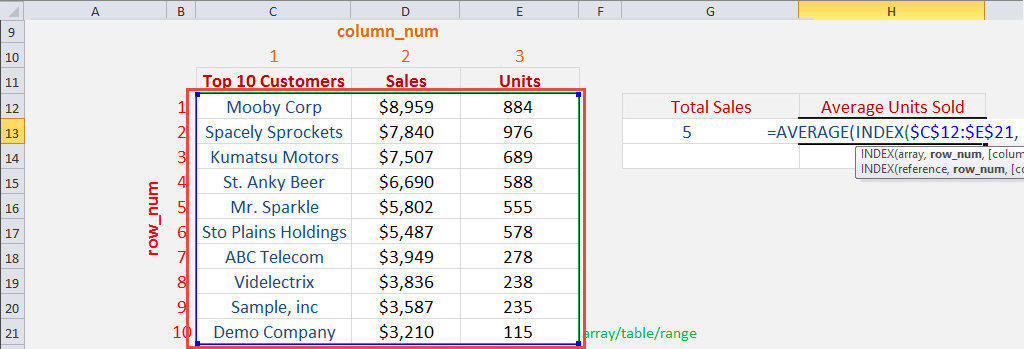## row_num

What is the row number we want to return?

We do not need to return the row, as we want to just average all of the units. Leave the row number blank:

## =AVERAGE(INDEX(\$C\$12:\$E\$21,,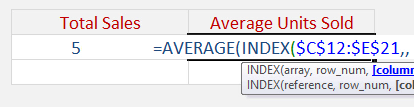## column_num

What is the column number we want to return?

Since we want the units column, this is column number 3. So place in 3:

## =AVERAGE(INDEX(\$C\$12:\$E\$21,,3))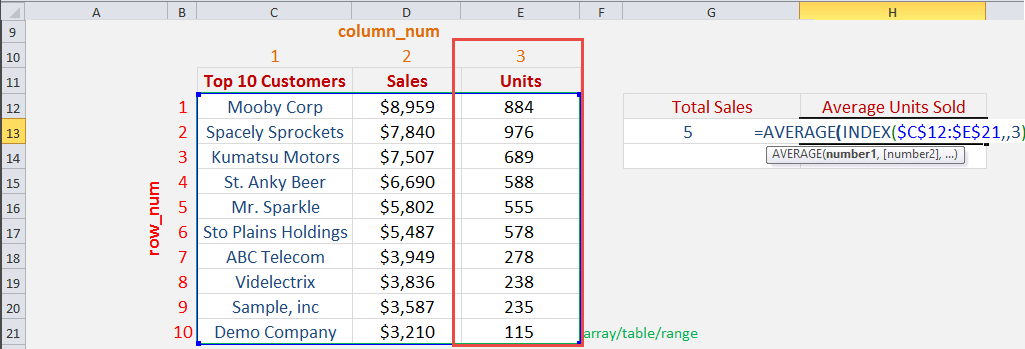Now you are able to get the Average Units Sold: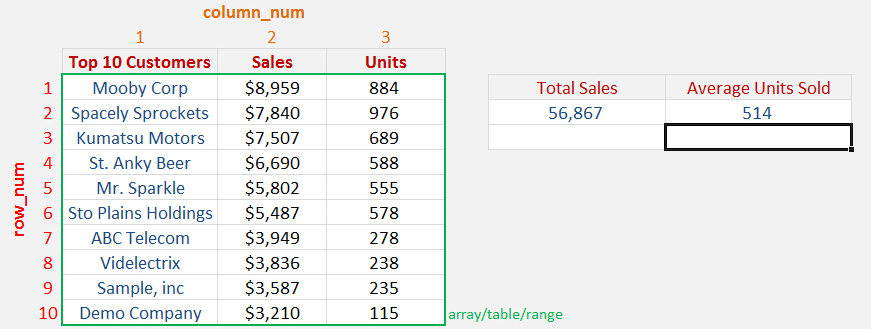## Index Match 2 Criteria with Data Validation

What does it do?

Searches the row position of a value/text in one column (using the MATCH function) and returns the value/text in the same row position from another column to the left or right (using the INDEX function)

Formula breakdown:

=INDEX(arrayMATCH(lookup_value, lookup_array, [match_type])

What it means:

=INDEX(return the value/textMATCH(from the row position of this value/text))

We can use the INDEX-MATCH formula and combine it with Data Validation drop down menus to return a value based on 2 criteria.

This is a little advanced so you will need to drop what you are doing and really focus.  Let’s go…

First we need to convert our data into an Excel Table by pressing Ctrl+T

See tutorial on how to convert to an Excel Table here

We then create drop down menus for our Sales Rep column and another one for our Units/Sales/Avg Sale column names

See tutorial on how to insert drop down menus here

Once the above are done we need to create our formula.

Download the workbook below to practice this method.

STEP 1:  We need to nest an INDIRECT function within the INDEX function and reference the Metric cell name (H14) with our Table name (Table1):

## =INDEX(INDIRECT(“Table1[“&H14&”]”),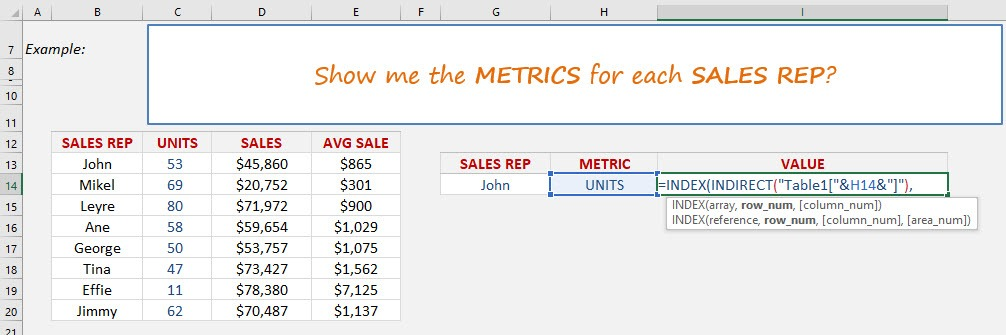This will give us our dynamic column name within the Excel Table.

STEP 2: We need to lookup our Sales Rep within the Sales Rep column table:

## MATCH(G14,Table1[SALES REP],0))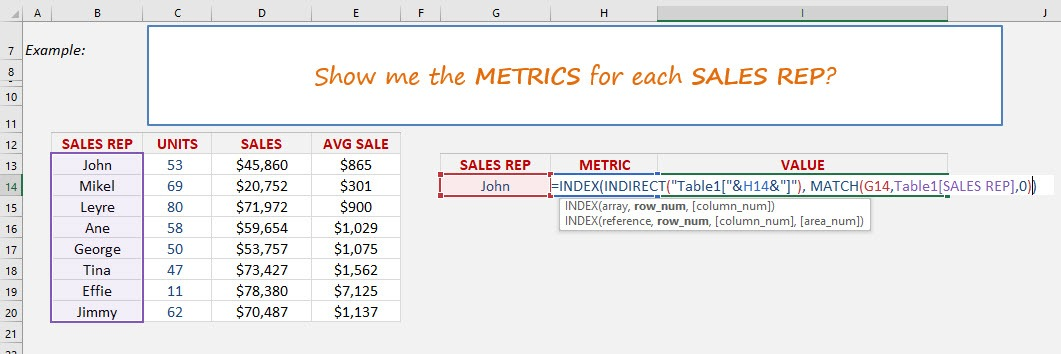So by combining these formulas we can choose two criteria (Sales Rep & Metric name) to return the respective value.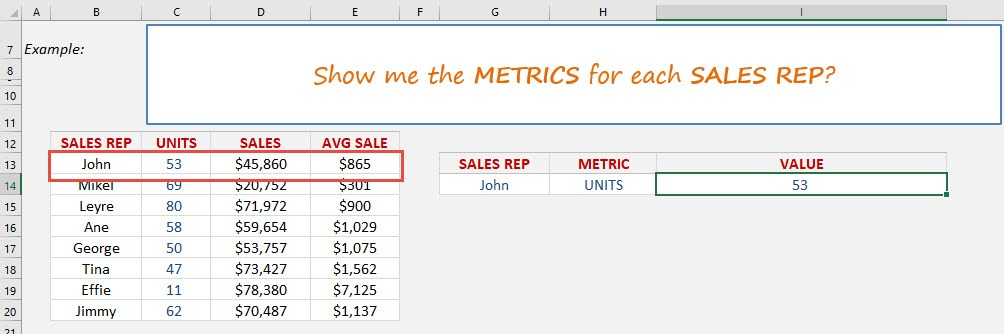## How To Use INDEX-MATCH Formula

What does it do?

Searches the row position of a value/text in one column (using the MATCH function)
and returns the value/text in the same row position from another column to the left or right (using the INDEX function)

Formula breakdown:

=INDEX(array, MATCH(lookup_value, lookup_array, [match_type])

What it means:

=INDEX(return the value/text, MATCH(from the row position of this value/text))

The VLOOKUP formula searches for a value in the first column of an array and returns a value to the right of that array.

How about if you wanted to return a value to the left hand side of that array?

Well, this is where the INDEX-MATCH formula comes in and gives you a helping hand!

It searches the row position of a value/text in one column (using the MATCH function) and returns the value/text in the same row position from another column to the left or right (using the INDEX function).

We want to get the stock id of the tablet, and we will use a combination of INDEX and MATCH to get this!

STEP 1: We need to enter the INDEX function in a blank cell:

## =INDEX(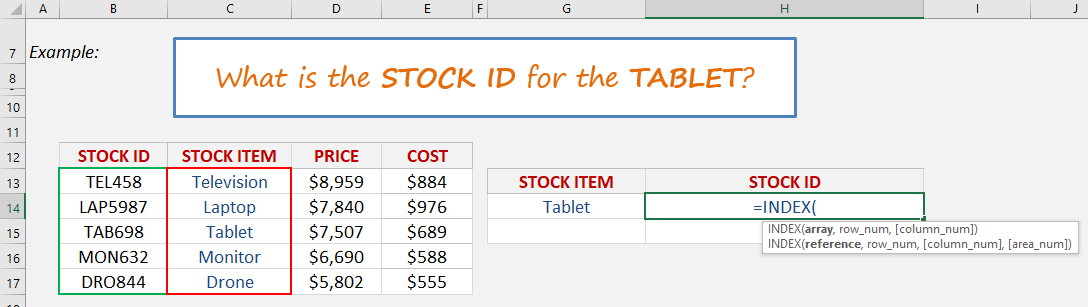STEP 2: The INDEX arguments:

## Array

Where is the list that contains the stock ids?

## =INDEX(B13:B17,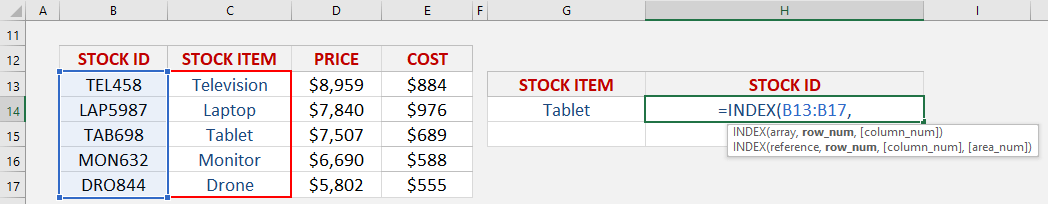## Row_num

What row number contains the data?

Let us use the Match function to get the row number.

## =INDEX(B13:B17, MATCH(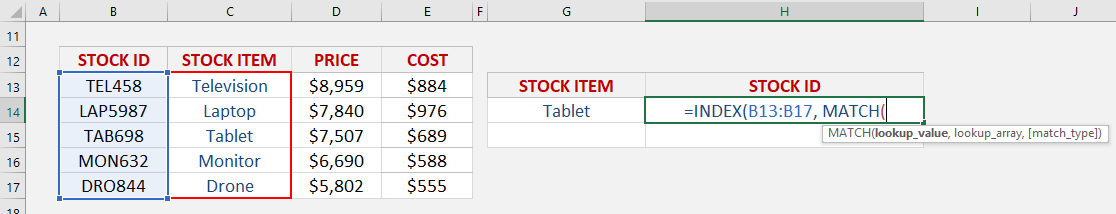STEP 3: The MATCH arguments:

## Lookup_value

What is the value that we want to match?

We want to match the Tablet.

## =INDEX(B13:B17, MATCH(G14,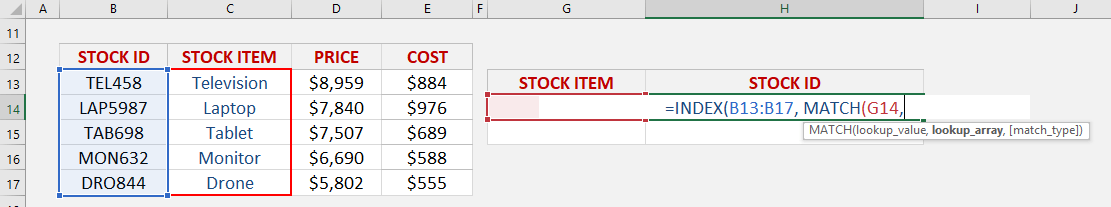## Lookup_array

Where is the list that contains the stock items?

## =INDEX(B13:B17, MATCH(G14, C13:C17,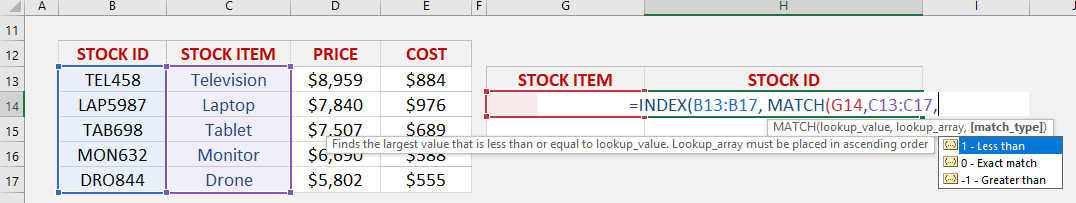## Match_type

What kind of matching do you want?

Let’s put in 0 to get the exact match

## =INDEX(B13:B17, MATCH(G14, C13:C17, 0))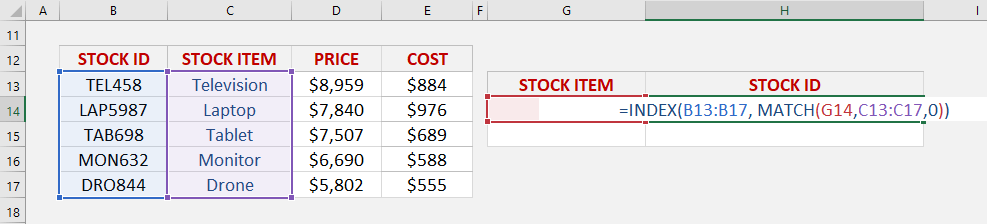With this, the MATCH function will get the row number containing the Tablet, which is row #3. Then with Row #3, we will get the stock id in that same row using the INDEX function.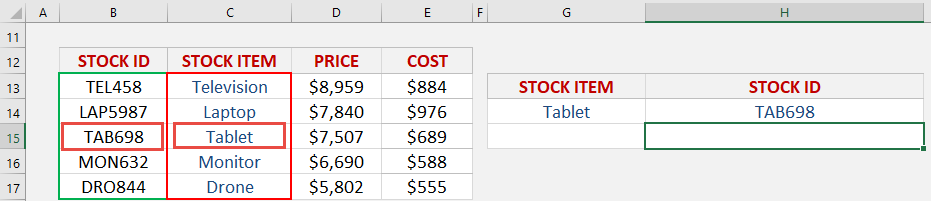Latest Tutorials

March 31, 2021

August 18, 2015

May 25, 2015